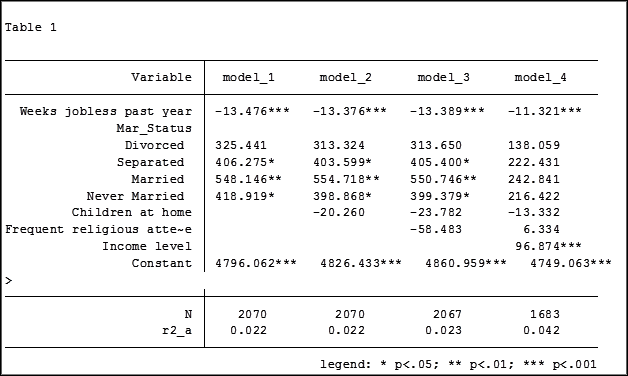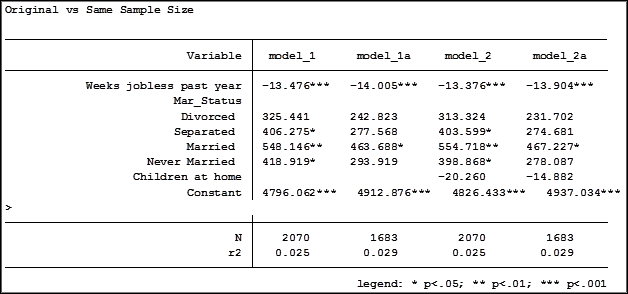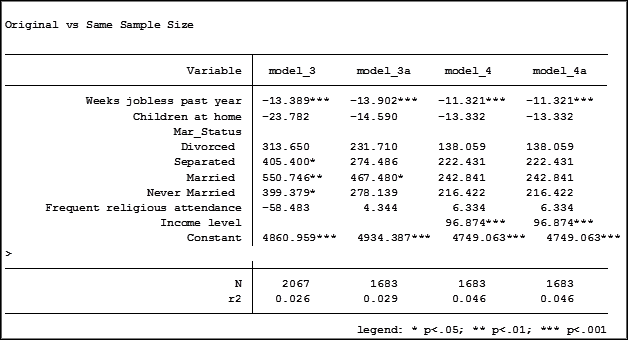# Using the Same Sample for Different Models in Stata

by Jeff MeyerIn my last article, Hierarchical Regression in Stata: An Easy Method to Compare Model Results, I presented the following table which examined the impact several predictors have on one’ mental health.At the bottom of the table is the number of observations (N) contained within each sample.

The sample sizes are quite large. Does it really matter that they are different? The answer is absolutely yes.

Fortunately in Stata it is not a difficult process to use the same sample for all four models shown above.

#### Some background info:

As I have mentioned previously, Stata stores results in temp files. You don’t have to do anything to cause Stata to store these results, but if you’d like to use them, you need to know what they’re called.

To see what is stored after an estimation command, use the following code:
ereturn list

After a summary command:
return list

One of the stored results after an estimation command is the function e(sample). e(sample) returns a one column matrix.  If an observation is used in the estimation command it will have a value of 1 in this matrix. If it is not used it will have a value of 0.

Remember that the “stored” results are in temp files.  They will disappear the next time you run another estimation command.

#### The Steps

So how do I use the same sample for all my models? Follow these steps.

Using the regression example on mental health I determine which model has the fewest observations.  In this case it was model four.

I rerun the model:
regress MCS  weeks_unemployed   i.marital_status   kids_in_house  religious_attend    income

Next I use the generate command to create a new variable whose value is 1 if the observation was in the model and 0 if the observation was not. I will name the new variable “in_model_4”.
gen in_model_4 = e(sample)

Now I will re-run my four regressions and include only the observations that were used in model 4. I will store the models using different names so that I can compare them to the original models.

My commands to run the models are:

regress MCS  weeks_unemployed   i.marital_status  if  in_model_4==1
estimates store model_1a

regress MCS  weeks_unemployed   i.marital_status   kids_in_house  if  in_model_4==1
estimates store model_2a

regress MCS  weeks_unemployed   i.marital_status   kids_in_house   religious_attend if  in_model_4==1
estimates store model_3a

regress MCS  weeks_unemployed   i.marital_status   kids_in_house  religious_attend    income if  in_model_4==1
estimates store model_4a

Note: I could use the code  if  in_model_4 instead of if  in_model_4==1. Stata interprets dummy variables as 0 = false, 1 = true.

Here are the results comparing the original models (eg. Model_1) versus the models using the same sample (eg. Model_1a):Comparing the original models 3 and 4 one would have assumed that the predictor variable “Income level” significantly impacted the coefficient of “Frequent religious attendance”. Its coefficient changed from -58.48 in model 3 to 6.33 in model 4.

That would have been the wrong assumption. That change is coefficient was not so much about any effect of the variable itself, but about the way it causes the sample to change via listwise deletion.  Using the same sample, the change in the coefficient between the two models is very small, moving from 4 to 6.

Jeff Meyer is a statistical consultant with The Analysis Factor, a stats mentor for Statistically Speaking membership, and a workshop instructor. Read more about Jeff here.Four Critical Steps in Building Linear Regression Models
While you’re worrying about which predictors to enter, you might be missing issues that have a big impact your analysis. This training will help you achieve more accurate results and a less-frustrating model building experience.